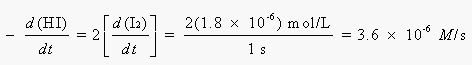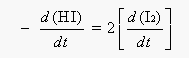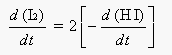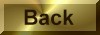Practice Problem 4

Calculate the rate at which HI disappears in the following reaction at the moment when I2 is being formed at a rate of 1.8 x 10-6 moles per liter per second:

2 HI(g)H2(g) + I2(g)

Solution

The balanced equation for the reaction shows that 2 moles of HI disappear for every mole of I2 formed.  Thus, HI is consumed in this reaction twice as fast as I2 is formed:Students sometimes get the wrong answer to this exercise because they become confused about whether the equation for the calculation should be written as:or as:You can avoid mistakes by checking to see whether your answer makes sense.  The balanced equation states that 2 moles of HI are consumed for every mole of I2 produced.  HI should therefore disappear (3.6 x 10-6 M/s) twice as fast as I2 is formed (1.8 x 10-6 M/s).